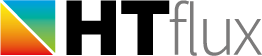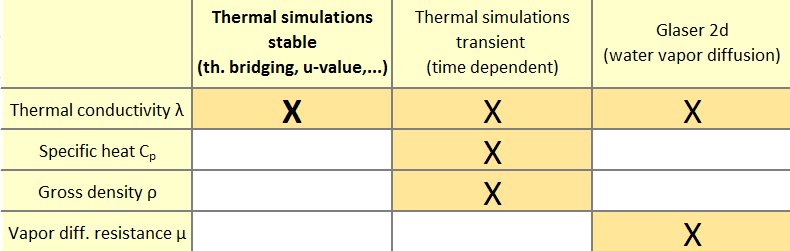# Physical properties of a material

## The thermal conductivity λ

The thermal conductivity of a material describes the ability of material to conduct heat. Most use materials use the constant model, where the thermal conductivity is described by a single constant value. The SI unit of the thermal conductivity is W/mK. Therefore a value of 1.0 means that, a temperature difference of 1 degree K (°C) applied to a 1 m thick piece of material will cause a heat flow with a power of 1 W.
There is also a more sophisticated model that allows you to define a temperature dependency of the conductivity. Another more sophisticated model allows the software to describe the heat conductivity of an air cavity according to ISO 6946 and ISO 10077-2.
(Note: HTflux can also be used in the imperial mode, mainly used in the US. In this case the conductivity will be described as resistivity with the IP-unit h.ft²/BTU.in)

It is necessary to provide correct values for the thermal conductivities for all type of simulations that can be processed with HTflux. Usually, you will have to use values listed in regulated documents or you will have to provide a certificate for using specific material values. This depends on your actual application and the local national regulations.

## The specific heat capacity C

The specific heat capacity or thermal capacity describes the ability of a material to store heat. Using SI units it is defined as the amount of heat (J) necessary to increase the temperature of 1 kg by one degree Kelvin (°C).
In HTflux it is necessary to provide a correct specific heat capacity value only for processing transient (time-dependent) simulations or for calculating dynamic performance figures (see U-value tool). In all other cases this parameter is not relevant for the calculation.

## The water vapor diffusion resistance factor µ

The water vapor resistance factor µ measures permeability of a material regarding water vapor diffusion. The number describes the resistance to vapor diffusion relative to the vapor diffusion of still air. It is therefore a ratio-number not having a specific unit.
Air having a µ value of 1 is very open to diffusion therefore low 1-digit values indicate a very “breathable” material. Concrete has a value of about 100 and plastics (PVC, PE) usually have values in the range of 10.000 to 100.000, whereas metal and glass is us usually absolutely tight to vapor diffusion. Usually a value of 1.000.000 is used in this case.  Often two values will be referenced, one for the wet state of the material and one for the dry state. You will have to choose the appropriate value in this case or use the value “on the safe side”.
It is only necessary to provide correct µ values in HTflux when using the Glaser 2d feature.

## The gross density ρ

The gross density measures the weight of a unit volume. In SI units it is the weight of a cube with lengths of 1m. The unit is therefore kg/m³.
In HTflux the gross density value is only required when performing transient simulations or calculation dynamic performance figures. (see u-value tool)

## What property is relevant for my simulation?